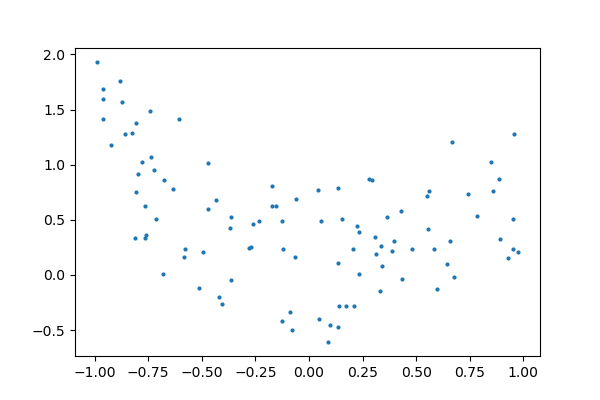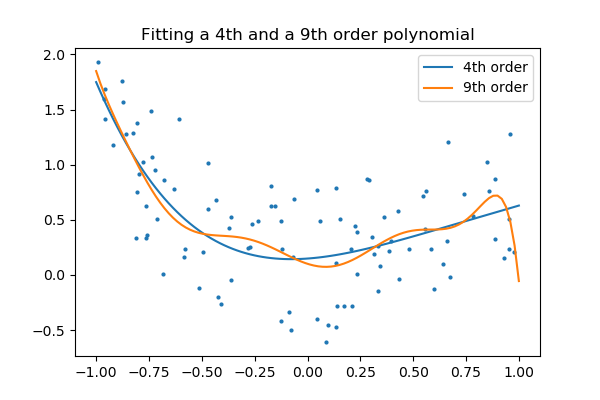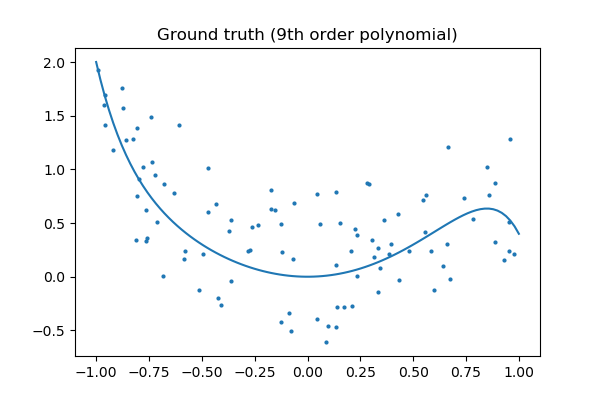# 3.6.10.10. Plot fitting a 9th order polynomial¶

Fits data generated from a 9th order polynomial with model of 4th order and 9th order polynomials, to demonstrate that often simpler models are to be prefered

```import numpy as np
from matplotlib import pyplot as plt
from matplotlib.colors import ListedColormap

from sklearn import linear_model

# Create color maps for 3-class classification problem, as with iris
cmap_light = ListedColormap(['#FFAAAA', '#AAFFAA', '#AAAAFF'])
cmap_bold = ListedColormap(['#FF0000', '#00FF00', '#0000FF'])

rng = np.random.RandomState(0)
x = 2*rng.rand(100) - 1

f = lambda t: 1.2 * t**2 + .1 * t**3 - .4 * t **5 - .5 * t ** 9
y = f(x) + .4 * rng.normal(size=100)

x_test = np.linspace(-1, 1, 100)
```

The data

```plt.figure(figsize=(6, 4))
plt.scatter(x, y, s=4)
```Fitting 4th and 9th order polynomials

For this we need to engineer features: the n_th powers of x:

```plt.figure(figsize=(6, 4))
plt.scatter(x, y, s=4)

X = np.array([x**i for i in range(5)]).T
X_test = np.array([x_test**i for i in range(5)]).T
regr = linear_model.LinearRegression()
regr.fit(X, y)
plt.plot(x_test, regr.predict(X_test), label='4th order')

X = np.array([x**i for i in range(10)]).T
X_test = np.array([x_test**i for i in range(10)]).T
regr = linear_model.LinearRegression()
regr.fit(X, y)
plt.plot(x_test, regr.predict(X_test), label='9th order')

plt.legend(loc='best')
plt.axis('tight')
plt.title('Fitting a 4th and a 9th order polynomial')
```Ground truth

```plt.figure(figsize=(6, 4))
plt.scatter(x, y, s=4)
plt.plot(x_test, f(x_test), label="truth")
plt.axis('tight')
plt.title('Ground truth (9th order polynomial)')

plt.show()
```Total running time of the script: ( 0 minutes 0.058 seconds)

Gallery generated by Sphinx-Gallery